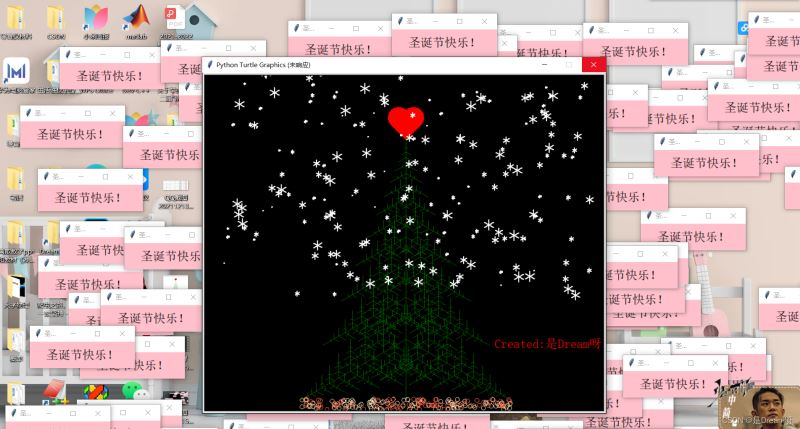﻿ Python绘制圣诞树+落叶+雪花+背景音乐+浪漫弹窗 五合一版圣诞树_python_脚本之家
python# Python绘制圣诞树+落叶+雪花+背景音乐+浪漫弹窗 五合一版圣诞树

## 一、背景故事## 二、五合一版圣诞树制作过程

```import turtle as t
import random
import pygame
import time
import tkinter as tk
```

### 1.基础圣诞树

```t.screensize(bg='black')  # 定义背景颜色，可以自己换颜色

def loving_heart(r):
l = 2 * r
t.left(45)
t.forward(l)
t.circle(r, 180)
t.right(90)
t.circle(r, 180)
t.forward(l)

# 树函数(递归)
def tree(d, s):
if d <= 0:
return
t.forward(s)
tree(d - 1, s * .8)
t.right(120)
tree(d - 3, s * .5)
t.right(120)
tree(d - 3, s * .5)
t.right(120)
t.backward(s)  # 回退函数

# 画爱心部分
t.penup()
t.goto(0, 200)  # 设置起点位置
t.pendown()
t.pencolor('red')  # 设置画笔颜色
t.color('red')
t.begin_fill()  # 对图形进行填充
loving_heart(20)  # 执行画爱心函数
t.end_fill()

# 画树部分
n = 100
t.speed(0)
# t.Turtle().screen.delay(0)
t.right(225)
t.color("dark green")
t.backward(n * 4.8)
tree(15, n)
t.backward(n / 5)

```

### 2.落叶效果

``` if random.randint(1, 2) == 1:
t.color('tomato')
else:
t.color('wheat')
```

```# 绘制落叶
for i in range(200):
a = 200 - 400 * random.random()
b = 10 - 20 * random.random()
t.speed(0)
t.up()
t.forward(b)
t.left(90)
t.forward(a)
t.down()
if random.randint(1, 2) == 1:
t.color('tomato')
else:
t.color('wheat')
t.circle(4)
t.up()
t.backward(a)
t.right(90)
t.backward(b)
```

### 3.雪花特效

```# 绘制雪花
def drawsnow():  # 定义画雪花的方法
t.speed(0)
t.ht()  # 隐藏笔头，ht=hideturtle
t.pensize(2)  # 定义笔头大小
for i in range(200):  # 画多少雪花
t.pencolor("white")  # 定义画笔颜色为白色，其实就是雪花为白色
t.pu()  # 提笔，pu=penup
t.setx(random.randint(-350, 350))  # 定义x坐标，随机从-350到350之间选择
t.sety(random.randint(-100, 350))  # 定义y坐标，注意雪花一般在地上不会落下，所以不会从太小的纵座轴开始
t.pd()  # 落笔，pd=pendown
dens = 6  # 雪花瓣数设为6
snowsize = random.randint(1, 10)  # 定义雪花大小
for j in range(dens):  # 就是6，那就是画5次，也就是一个雪花五角星
# t.forward(int(snowsize))  #int（）取整数
t.fd(int(snowsize))
t.backward(int(snowsize))
# t.bd(int(snowsize))  #注意没有bd=backward，但有fd=forward，小bug
t.right(int(360 / dens))  # 转动角度
drawsnow()
```

### 4.背景音乐

```file=r'薛之谦 - 迟迟.mp3'		# 音乐的路径
pygame.mixer.init()						# 初始化
pygame.mixer.music.play()				# 开始播放音乐
```

### 5.浪漫弹窗

```# 弹窗制作
def dow():
window = tk.Tk()
width = window.winfo_screenwidth()
height = window.winfo_screenheight()
a = random.randrange(0, width)
b = random.randrange(0, height)
window.title('圣诞节快乐')
window.geometry("200x50" + "+" + str(a) + "+" + str(b))
tk.Label(window,
text='圣诞节快乐！',  # 标签的文字
bg='pink',  # 背景颜色
font=('..', 17),  # 字体和字体大小
width=18, height=2  # 标签长宽
).pack()  # 固定窗口位置
window.mainloop()

for i in range(100):  # 需要的弹框数量
time.sleep(0.01)
```

### 6.署名制作

```# 写下署名
t.color("red")  # 填充颜色
t.up()  # 抬笔
t.goto(170,-200)
t.down()  # 落笔
t.write("Created:是Dream呀", font=("宋体", 18, "normal"))
t.ht()
# t.done()
t.hideturtle()

```

## 三、源码分享

```import turtle as t
import random
import pygame
import time
import tkinter as tk

file=r'薛之谦 - 迟迟.mp3'		# 音乐的路径
pygame.mixer.init()						# 初始化
pygame.mixer.music.play()				# 开始播放音乐

t.screensize(bg='black')  # 定义背景颜色，可以自己换颜色

def loving_heart(r):
l = 2 * r
t.left(45)
t.forward(l)
t.circle(r, 180)
t.right(90)
t.circle(r, 180)
t.forward(l)

# 树函数(递归)
def tree(d, s):
if d <= 0:
return
t.forward(s)
tree(d - 1, s * .8)
t.right(120)
tree(d - 3, s * .5)
t.right(120)
tree(d - 3, s * .5)
t.right(120)
t.backward(s)  # 回退函数

# 画爱心部分
t.penup()
t.goto(0, 200)  # 设置起点位置
t.pendown()
t.pencolor('red')  # 设置画笔颜色
t.color('red')
t.begin_fill()  # 对图形进行填充
loving_heart(20)  # 执行画爱心函数
t.end_fill()

# 画树部分
n = 100
t.speed(0)
# t.Turtle().screen.delay(0)
t.right(225)
t.color("dark green")
t.backward(n * 4.8)
tree(15, n)
t.backward(n / 5)

# 绘制落叶
for i in range(200):
a = 200 - 400 * random.random()
b = 10 - 20 * random.random()
t.speed(0)
t.up()
t.forward(b)
t.left(90)
t.forward(a)
t.down()
if random.randint(1, 2) == 1:
t.color('tomato')
else:
t.color('wheat')
t.circle(4)
t.up()
t.backward(a)
t.right(90)
t.backward(b)
# 绘制雪花
def drawsnow():  # 定义画雪花的方法
t.speed(0)
t.ht()  # 隐藏笔头，ht=hideturtle
t.pensize(2)  # 定义笔头大小
for i in range(200):  # 画多少雪花
t.pencolor("white")  # 定义画笔颜色为白色，其实就是雪花为白色
t.pu()  # 提笔，pu=penup
t.setx(random.randint(-350, 350))  # 定义x坐标，随机从-350到350之间选择
t.sety(random.randint(-100, 350))  # 定义y坐标，注意雪花一般在地上不会落下，所以不会从太小的纵座轴开始
t.pd()  # 落笔，pd=pendown
dens = 6  # 雪花瓣数设为6
snowsize = random.randint(1, 10)  # 定义雪花大小
for j in range(dens):  # 就是6，那就是画5次，也就是一个雪花五角星
# t.forward(int(snowsize))  #int（）取整数
t.fd(int(snowsize))
t.backward(int(snowsize))
# t.bd(int(snowsize))  #注意没有bd=backward，但有fd=forward，小bug
t.right(int(360 / dens))  # 转动角度
drawsnow()
# 写下署名
t.color("red")  # 填充颜色
t.up()  # 抬笔
t.goto(170,-200)
t.down()  # 落笔
t.write("Created:是Dream呀", font=("宋体", 18, "normal"))
t.ht()
# t.done()
t.hideturtle()

time.sleep(2)

# 弹窗制作
def dow():
window = tk.Tk()
width = window.winfo_screenwidth()
height = window.winfo_screenheight()
a = random.randrange(0, width)
b = random.randrange(0, height)
window.title('圣诞节快乐')
window.geometry("200x50" + "+" + str(a) + "+" + str(b))
tk.Label(window,
text='圣诞节快乐！',  # 标签的文字
bg='pink',  # 背景颜色
font=('..', 17),  # 字体和字体大小
width=18, height=2  # 标签长宽
).pack()  # 固定窗口位置
window.mainloop()